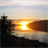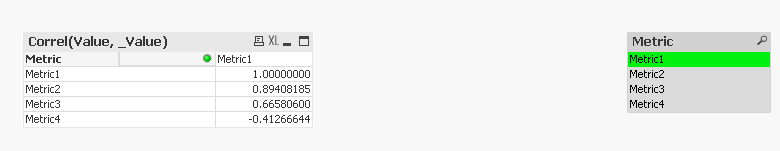# QlikView App Dev

Discussion Board for collaboration related to QlikView App Development.

Announcements
cancel
Showing results for
Did you mean:Creator II

## Dynamically finding correlations

Hello all,

I'm trying to figure out a way to dynamically show correlations between metrics.  The metric names are grouped together in 1 field with another field showing the values.  I'm changed this table in the script so that each metric is now it's own field associated with a value.  I've kept the original table as well.

I'm trying to use the Correl function to show correlations between each metrics, however, there are nearly 80 metrics so making a correl function for each combination isn't really feasible.  (ex: Correl(Metric1,Metric2), Correl(Metric1,Metric3),  Correl(Metric2,Metric3), etc.)

Is there a way to make it dynamic to show correlation percentages for a selected metric?  I.E. if Metric1 is selected, the chart would show all correlation values for Metric1)

Here's an example of the tables:

My original table looks like this:

AccountMetricValue
Account1Metric1832
Account1Metric21032
Account1Metric3213
Account2Metric13213
Account2Metric23432
Account2Metric37689
Account3Metric11034
Account3Metric21340
Account3Metric38903

I took this table and pivoted it in the script so that I have a 2nd table like this:

AccountMetric1Metric2Metric3
Account18321032213
Account2321334327689
Account3103413408903
1 Solution

Accepted SolutionsMVP

Actually try this:

If(GetSelectedCount(Metric) > 0, Correl({<_Metric = e(Metric)>}Value, _Value), Correl(Value, _Value))

8 RepliesMVP

Would you be able to share what you have? In like a qvw file and may be we can take it forward? and also share some information about the expected output?Creator II
Author

Thank you for the reply Sunny.

I'm working on getting a sample QVW up. The expected output would be to have the metrics as the dimension (all metrics except the one selected) with the correlation percentage as the expression.

So for instance, if Metric1 is selected, the output would be something like this:

MetricCorrelation
Metric280%
Metric3

50%

Metric43%
Metric568%

Edit:

Or if Metric2 is selected:

MetricCorrelation
Metric180%
Metric341%
Metric423%
Metric569%Creator II
Author

I've attached a sample QVWMVP

This is what you need?No selectionCreator II
Author

I feel like it's very close.

When you select a metric though I would not want to show that same metric in the dimension.  For instance when you select Metric1, Metric1 would not appear on the chart since it will always have a correlation with itself if that makes sense.

I also don't want to use conditional shows for expressions since I will have so many combinations, it will be incredibly cumbersome.MVP

Try this:

Correl({<_Metric = e(Metric)>}Value, _Value)MVP

Actually try this:

If(GetSelectedCount(Metric) > 0, Correl({<_Metric = e(Metric)>}Value, _Value), Correl(Value, _Value))Creator II
Author

That works!  Thank you so much Sunny!!  You saved me a lot of script load time as well by eliminating the reverse-crosstable!